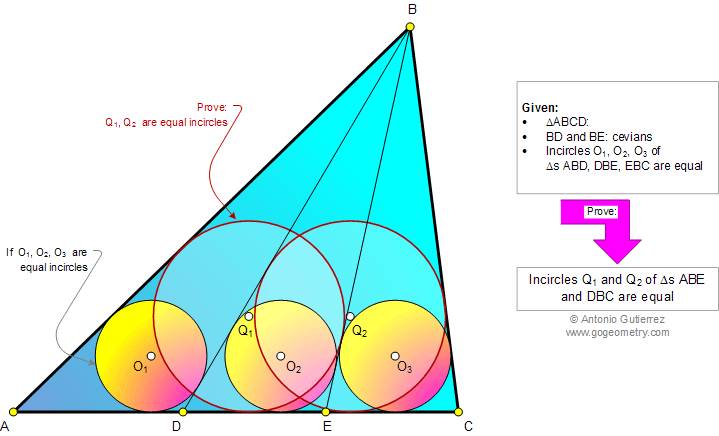# Online Geometry Problem 935: Infographic, Triangle, Cevian, Equal Incircles, Inscribed Circles. Level: High School, SAT Prep, College, Mathematics Education

< PREVIOUS PROBLEM  |  NEXT PROBLEM >

 The infographic below shows a triangle ABC with cevians BD and BE. If the incircles O1, O2, and O3 of the triangles ABD, DBE, and EBC, respectively, are equal, prove that the incircles Q1 and Q2 of the triangles ABE and DBC are equal. A cevian is a line segment with one endpoint on a vertex of a triangle and the other endpoint on the opposite side.Home | Search | Geometry | Problems | All Problems | Open Problems | Visual Index | 10 Problems | 931-940 | Equal Incircles with Animation | Triangle | Incircle | Angle Bisector | Circle | Email | Solution / comment | By Antonio Gutierrez